## Graph Theory: Part I (Introduction)

by Jesse Farmer on Tuesday, April 15, 2008

This is the first in a multi-part series about graph theory here on 20bits. This started out of me wanting to write about some of the mathematical aspects of Facebook, but I realized that many people might not have a sufficient background to just jump right in. Rather than cover the all the ground in one article I decided to break it up into multiple parts. This is the first part, a quick introduction to graph theory.

Graph theory is a fundamental area of study in discrete mathematics. As the name implies graph theory is about graphs, so I'll first define graph and then discuss why people are so interested in studying these critters. I'm going to assume you're familiar with the idea of ordered pairs and sets.

### Some Definitions

Definition. A graph G is a pair (V,E) of sets, called the vertex set and edge set. V is a collection of abstract vertices, written {v1, v2,...,vn} and E is a collection of ordered pairs of vertices, called edges.

As you can see, this is pretty abstract. The definition leaves you free to define both what the vertices and edges are, precisely. Vertices could be cities and edges could be interstate highways. An example: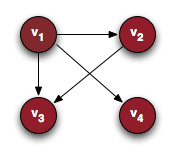This type of graph is called a directed graph because some of the edges have a direction, i.e., they only go one way. Going back to the original definition, we have V = {v1,v2,v3,v4} and E = {(v1,v2),(v1,v3),(v1,v4),(v2,v3)}

Definition. Let G be a graph, then we write V(G) to mean the vertex set of G and E(G) to mean the edge set. We will just write V and E if the context makes it clear which graph we're talking about.

Definition. Let G be a graph and let u,v ∈ V(G). We write v ~ u if there is an edge connecting v to u, i.e., if (v,u) ∈ E(G).

Sometimes we don't care about direction and can make edges directionless. These sorts of graphs are called undirected graphs and look like this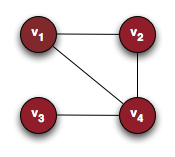Definition. A graph G is an undirected graph if u ~ v implies v ~ u for all u,v ∈ V(G).

### Concrete examples

I'd be remiss if I kept talking like graph theory is some pie-in-the-sky theoretical abstraction. In fact, many real-world situations can be modeled using graph theory. Some examples:

• Shipping routes

The vertices are shipping hubs and the edges are the routes between them

• Social networks

The vertices are people and the edges are social connections (e.g., p ~ q is p is a friend of q)

• Telecommunications networks

The vertices are computers on the network and the edges are the network connections between them

• Disease transmission

The vertices are organisms which can carry the disease and the edges represent one organism spreading it to another

• Sexual networks

The vertices are people and the edges denote which pairs have slept together (see, e.g., The Structure of Romantic and Sexual Relations at Jefferson High)

### More Definitions

Essentially any situation where you want to consider pairs of objects and the connections between those pairs can be analyzed using graph theory. There are a few more definitions to cover.

Definition. A graph loop or just loop is a vertex v which is connected to itself, i.e., v ~ v. A graph with no such loops is called a simple graph.

Note. Some authors allow multiple edges between vertices. In this situation graphs with no loops and at most one edge between any two vertices is called a simple graph. Although this type of graph is less than ideal for analysis it occurs relatively frequently in reality, e.g., two different roads connecting a pair of cities or redundant network connections. I'll make sure to note where we are dealing with such graphs and how we work around it.

Definition. Let G be a graph. A path or a walk is a collection of vertices v1, v2, ..., vk} such that vi ~ vi+1 for all i, 1 ≤ i < k. A path with no repeated vertices is called simple, and a path such that vk ~ v1 is called a closed path, closed walk, or a cycle.

Definition. A weighted graph G is a graph such that each edge in E(G) has an associated weight, typically a real number.

Weighted graphs are the stuff of many famous algorithms. There are a whole slew of algorithms dedicated to finding the shortest path between two vertices in a weighted graph, where "shortest" means the path with the smallest weight. The algorithms vary in performance depending on several factors: the ratio of vertices to edges, whether the graph has negative weights, whether we have a good heuristic for determining what a path might cost, etc.

### Important Graphs

There are some graphs every student of computer science or discrete mathematics is just sort of expected to know.

##### The Complete Graph

The complete graph on n vertices, written Kn, has n vertices and an edge for every pair of distinct vertices. K4 looks like this, for example: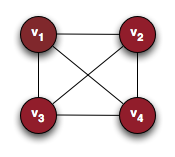##### The Cycle

The cycle of n vertices, written Cn is the undirected graph on n vertices which consists of precisely one cycle containing every vertex. C4 looks like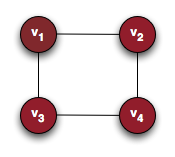##### The Complete Bipartite Graph

The complete bipartite graph, written Kn,m is an undirected graph that has the union of two disjoint sets of size n and m, respectively, as its vertex set, i.e., V(Kn,m) = V ∪ U, V ∩ U = ∅, |V| = n, and |U| = m. Its edges satisfy the property that v ~ u for all v ∈ V and all u ∈ U. It's easier to draw than to write, I think, so here is K2,2: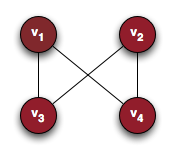Here V = {v1,v2} and U = {v3, v4}. Any graph G whose vertex set V(G) can be partitioned into two sets such that no two vertices in a partition share an edge is called bipartite, meaning "of two parts." The complete bipartite graph is the bipartite graph which has as many edges as possible without ceasing to be bipartite.

### Conclusion

That's it for the basics. Hopefully you understand what a graph is, some of the problems graph theory is useful in analyzing, and some of the common constructs in graph theory. In Part II I'm going to cover the relationship between graph theory and linear algebra. My ultimate plan is to use this relationship to come up with a way to rank people's influence in social networks, using a measure called eigenvector centrality.

### Errata

I left out a few definitions yesterday, so here they are.

Definition. Let G be a graph and let v ∈ V(G) be a vertex. The out degree of v, written deg-(v), is the number of edges directed away from v. Conversely, the in degree of v, written deg+(v), is the number of edges directed towards v. If G is undirected then deg+(v) = deg-(v) for all v ∈ V(G), so we call this number the degree of v and write it deg(v).

The plus (+) and minus (-) have to do with the idea of flow. If you imagine a directed graph G and some substance diffusing through the graph along the edges then the out degree and the in degree measure the degree to which that vertex loses (-) or accumulates (+) the substance, respectively.